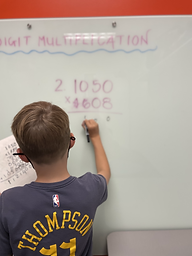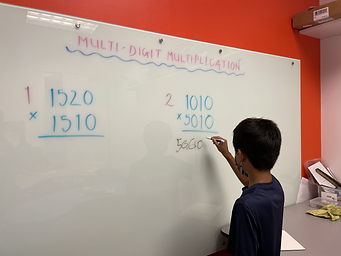## Mr. Jake

### Target 1​

###### Lesson Type:

New

Measurment

:

Techniques and Formulas

Solve problems involving area.

###### 1:

Use previous area understandings (of rectangular figures) to gain insight into the area of new geometric shapes.

###### 2:

Find the area of triangles, trapezoids, and parallelograms.

###### 3:

Determine the algebraic equations for calculating the area of a triangle (½ base * height), a trapezoid (½ height * (base1 + base2)), and a parallelogram (base * height).

###### 4:

Calculate the area of a shaded region of a figure.

7th

###### Vocabulary:

Area, Triangle, Base, Height

Activities:

Students reviewed multiplication with multiple digits.

Students created their own triangles on a grid and solved for the area of one another's drawings.

Students completed workbook pages to reinforce what they learned.### Home Exploration

###### Guiding Questions:## Absent Students:

Hudson

### Target 2

:

###### Vocabulary:

Activities:### Home Exploration

###### Guiding Questions:### Target 3

:

###### Vocabulary:

Activities:### Home Exploration